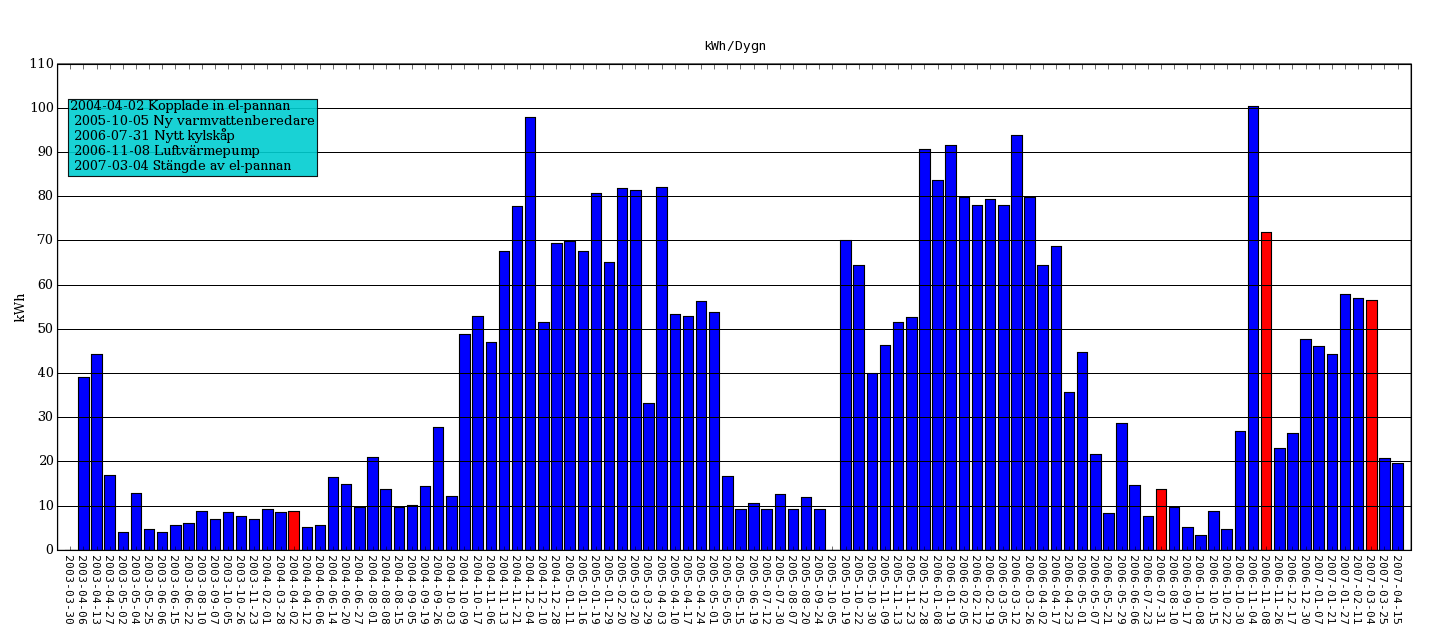# ugly code

Hi

I'm using matplotlib for producing a bar-chart displaying my
electricity consumption. One thing that really annoys me is
that generally python code is quite visually appealing, the
same goes for the code in the matplotlib-examples.
The code I have produced here is frankly quite ugly
when it comes to setting all the properties I want.
Is there a smarter way of doing this, perhaps using dictionaries etc?
(I have attached the genereated png-file)

cheers

//Fredrik···

--

from parser_el import *
from pylab import *

# Override some matplotlibrc defaults
# to get some nicer plots
rc('figure', figsize=(18,8))
rc('figure.subplot', bottom=0.14)
rc('figure.subplot', left=0.04)
rc('figure.subplot', right=0.98)

p = parser_el()
N = len(p.dates)
ind = arange(N)
p1 = bar(ind, p.kwh_avg, color='b', align='center')

# change color of important bars...
for i in p.info:
if type(i)==datetime:
index = p.dates.index(i)
if index:
p1[index].set_facecolor('r')

# Set axis, grid properties & use dates as xticks
title('kWh/Dygn')
ylabel('kWh')
axis([-1,N,0,110])
locs, labels = xticks(ind, p.dates_string)
setp(labels, 'rotation', '270', 'ha', 'center', fontsize=10,
family='monospace')
xgridlines = getp(gca(), 'xgridlines')
ygridlines = getp(gca(), 'ygridlines')
setp(ygridlines, 'linestyle', '-')
setp(xgridlines, 'linestyle', 'None')
ax = subplot(111)
ax.yaxis.set_major_locator(MultipleLocator(10)) # Set y-gridlines
multiples of 10
grid(True)

text(0,85, p.info_string, bbox=dict(facecolor='darkturquoise', alpha=0.9))

# Store graph as image
savefig('el', dpi=80, orientation='landscape', format='png')

show()

=

--

One thing that really annoys me is that generally python
code is quite visually appealing

Tastes must vary!
What is your point of comparison??

Controlling may graph properties is never going to be
beautiful. But why not define a class that can build
a figure with your desired defaults?

# change color of important bars...
for i in p.info:
if type(i)==datetime:
index = p.dates.index(i)
if index:
p1[index].set_facecolor('r')

Apparently p.dates is NOT a list?
(You seem to assume None is returned if a ValueError would be raised.)
Anyway, maybe something like:

colorindices = (p.dates.index(d) for d in p.info if d in p.dates)
for index in colorindices:
p1[index].set_facecolor('r')

Cheers,
Alan Isaac

···

On Sun, 22 Apr 2007, Hans Strotzer apparently wrote:

You might consider using the object oriented part of matplotlib instead
of the matlab style of doing things. I tend to make my plots a plot()
method of whatever kind of analysis class I've created:

from pylab import figure

class Analysis(object):
def init(self):
self.x = range(10)
self.y = range(10)
def plot(self):
self.f = figure() # create a figure
self.a = self.f.add_subplot(111) # create axes
self.a.set_xlabel('time (s)')
self.a.set_ylabel('value')
self.a.plot(self.x, self.y)

a = Analysis()
a.plot()

Cheers,

Martin

Hans Strotzer wrote:

···

Hi

I'm using matplotlib for producing a bar-chart displaying my
electricity consumption. One thing that really annoys me is
that generally python code is quite visually appealing, the
same goes for the code in the matplotlib-examples.
The code I have produced here is frankly quite ugly
when it comes to setting all the properties I want.
Is there a smarter way of doing this, perhaps using dictionaries etc?
(I have attached the genereated png-file)

cheers

//Fredrik
--

from parser_el import *
from pylab import *

# Override some matplotlibrc defaults
# to get some nicer plots
rc('figure', figsize=(18,8))
rc('figure.subplot', bottom=0.14)
rc('figure.subplot', left=0.04)
rc('figure.subplot', right=0.98)

p = parser_el()
N = len(p.dates)
ind = arange(N)
p1 = bar(ind, p.kwh_avg, color='b', align='center')

# change color of important bars...
for i in p.info:
if type(i)==datetime:
index = p.dates.index(i)
if index:
p1[index].set_facecolor('r')

# Set axis, grid properties & use dates as xticks
title('kWh/Dygn')
ylabel('kWh')
axis([-1,N,0,110])
locs, labels = xticks(ind, p.dates_string)
setp(labels, 'rotation', '270', 'ha', 'center', fontsize=10,
family='monospace')
xgridlines = getp(gca(), 'xgridlines')
ygridlines = getp(gca(), 'ygridlines')
setp(ygridlines, 'linestyle', '-')
setp(xgridlines, 'linestyle', 'None')
ax = subplot(111)
ax.yaxis.set_major_locator(MultipleLocator(10)) # Set y-gridlines multiples of 10
grid(True)

text(0,85, p.info_string, bbox=dict(facecolor='darkturquoise', alpha=0.9))

# Store graph as image
savefig('el', dpi=80, orientation='landscape', format='png')

show()

=

------------------------------------------------------------------------

------------------------------------------------------------------------

-------------------------------------------------------------------------
This SF.net email is sponsored by DB2 Express
Download DB2 Express C - the FREE version of DB2 express and take
control of your XML. No limits. Just data. Click to get it now.
http://sourceforge.net/powerbar/db2/

------------------------------------------------------------------------

_______________________________________________
Matplotlib-users mailing list
Matplotlib-users@lists.sourceforge.net
https://lists.sourceforge.net/lists/listinfo/matplotlib-users